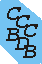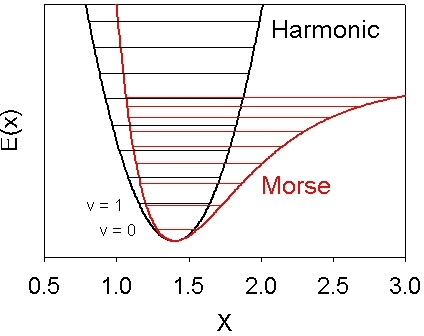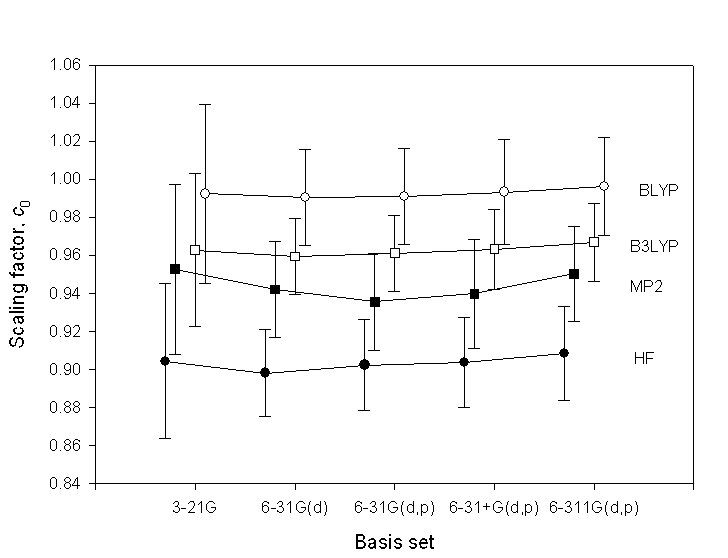National Institute of Standards and Technology
 Computational Chemistry Comparison and Benchmark DataBase Release 22 May 2022 NIST Standard Reference Database 101
 I Introduction II Experimental data III Calculated data IV Data comparisons V Cost comparisons VI Input and output files VII Tutorials and Units VIII Links to other sites IX Feedback X Older CCCBDB versions XII Geometries XIII Vibrations XIV Reaction data XV Entropy data XVI Bibliographic data XVII Ion data XVIII Bad calculations XIX Index of properties XX H-bond dimers XXI Oddities

NIST policy on privacy, security, and accessibility.

The National Institute of Standards and Technology (NIST) is an agency of the U.S. Department of Commerce.# Vibrational scaling factors

The vibrational frequencies produced by ab initio programs are often multiplied by a scale factor (in the range of 0.8 to 1.0) to better match experimental vibrational frequencies. This scaling compensates for two problems:
1) The electronic structure calculation is approximate. Usually less than a relativistic full configuration interaction is performed.
2) The potential energy surface is not harmonic. For bond stretches a better description of the potential energy surface is given by the Morse potential E(x) = D(1-exp(-β( x-x0 )))2 illustrated below. In this equation E is the potential energy, D, β, and x0 are constants, and x is the interatomic distance.The programs that predict vibrational frequencies do so by calculating the second derivative of the potential energy surface with respect to the atomic coordinates. This provides the curvature at the bottom (minimum) of the well. For a harmonic potential E(x) = k x 2 this is directly related to the vibrational energy level spacing. For a Morse potential (with the same second derivative at the minimum) the anharmonicity causes the vibrational energy levels to be more closely spaced as illustrated in the figure.

Experimentally usually the fundamental (v=0 to v=1 energy) is measured. If enough vibrational levels are observed a harmonic frequency can be estimated with a formula such as G(v) = ωe(v+1/2) - ωexe(v+1/2)2 + ωeye(v+1/2)3 + ... where G(v) is the vibrational energy, v is the vibrational quantum number, ωe is the harmonic frequency, and ωexe and ωeye are anharmonic constants. For polyatomic molecules usually only the fundamental is experimentally observed.

## How we calculate the vibrational scaling factors

We use the experimentally observed vibrational frequencies (νi), and the theoretical vibrational frequencies (ωi).
The scaling factor (c) and its relative uncertainty (ur) are obtained from the following sums over the vibrational frequencies:

c = Σ(νiωi)/ Σ(ωi2)
ur2 = (Σ(ωi2 • (c-νi/ωi)2))/(Σ(ωi2))

Try out the CCCBDB pages for calculating vibrational scaling factors.

## How much do the factors change with basis set?

The following figure shows several scaling factors and their uncertainties. The figure is from: K. K. Irikura, R. D. Johnson III, R. N. Kacker, J. Phys. Chem. A., 2005, 109(37), 8430-8437.
Filled cirlces = HF, filled squares = MP2, open cirlces = BLYP, open squares = B3LYP.The scaling factors depend weakly on basis set. The uncertainties are about twice as large for the 3-21G basis set as for the other basis sets, all of which include polarization functions. This suggests that polarization functions are important for avoiding markedly poor predictions of vibrational frequencies. For larger basis sets the scaling factors and uncertainties have non-significant changes. Thus the same scaling factors can be used for other basis sets.

See the pages listing vibrational scaling factors: III.B.3.a Precomputed vibrational scaling factors.